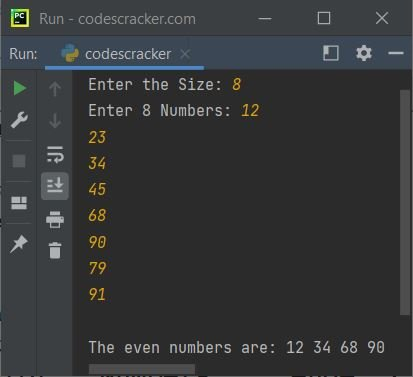# Python yield Keyword

The yield keyword in Python is similar to return, except that it returns a generator.

A generator is similar to an iterator, except that we can only iterate over once. Because generators don't store all values in the memory, rather they generate values on the fly. For example:

```num = 2
mygen = (2*i for i in range(1, 11))
for x in mygen:
print(x)```

The output is:

```2
4
6
8
10
12
14
16
18
20```

which is basically the table of 2. If you try to access the elements of mygen generators again, then you'll get nothing, as generators can only be used once.

In above program, you can consider the following code:

`range(1, 11)`

as

`[1, 2, 3, 4, 5, 6, 7, 8, 9, 10]`

Just like list comprehension, the generators can be created. The only difference is, we have to use () instead of []. Also we can not perform for x in mygen for second time, since they can only be used at once.

Therefore, the following program:

```mylst = [num*num for num in range(5)]
for x in mylst:
print(x)
print("----------")
for x in mylst:
print(x)```

produces:

```0
1
4
9
16
----------
0
1
4
9
16```

whereas the following program:

```mygen = (num*num for num in range(5))
for x in mygen:
print(x)
print("----------")
for x in mygen:
print(x)```

produces:

```0
1
4
9
16
----------```

Now let's come back to the actual topic, the yield keyword. I've defined generator, because it is important, while talking about, yield keyword. As any function contains a yield keyword is termed as a generator.

The yield keyword is used when we need to return from a function, but without destroying the states of local variable. Also the execution must start from the last yield statement, when the function is called. For example:

```def codescracker():
yield 10
yield 20
yield 30
yield 40
yield 50

for x in codescracker():
print(x)```

The output is:

```10
20
30
40
50```

The above program can also be created in this way:

```def codescracker():
for i in range(10, 51, 10):
yield i

for x in codescracker():
print(x)```

Note - The range() function generates a sequence of numbers.

Here is the last example program, demonstrating the yield keyword in Python. This program allows user to define the size of list, along with all its numbers (elements), to find and print all even values:

```def even(mylist):
for val in mylist:
if val%2 == 0:
yield val

mylist = []
print("Enter the Size: ", end="")
tot = int(input())
print("Enter", tot, "Numbers: ", end="")
for i in range(tot):
num = int(input())
mylist.append(num)

print("\nThe even numbers are: ", end="")
for x in even(mylist):
print(x, end=" ")```

The snapshot given below shows the sample run of above program, with user input 8 as size, 12, 23, 34, 45, 68, 90, 79, 91 as eight numbers:Python Online Test

« Previous Tutorial Next Tutorial »